# Far Off-Axis Conic Surfaces for Non-Rotationally Symmetric Surface Generation

Non-rotationally symmetric surfaces are produced by the ability to servo a tool in a coordinated manner, with a workpiece rotating on a two-axis lathe. The diamond machining of far off-axis segments for use in large mosaic mirrors is one main application of this technology. The generation of an off-axis mirror segment in a non-rotationally symmetric manner enables the diamond turning lathes to be sized into mirror segment dimensions, without encompassing the full axis-to-segment distance.

This article describes these far off-axis mirror surfaces in a manner ideal for the generation of non-rotationally symmetric surfaces.

## Description of Off-Axis Conic Surfaces

The aspheric optical surfaces are represented using the most common sagitta equation as follows: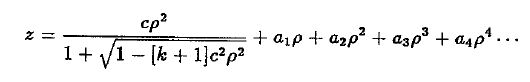The equation describes a conic surface of revolution, which is altered with simple polynomial terms in a cylindrical coordinate system. The section of this non-rotationally symmetric surface is generally represented by a transcendential equation. Most of the large mosaic mirrors are expected to be unmodified conic surfaces of revolution.

Thompson reported the description of a paraboloid case with orientation limitations. Thompson's derivation was followed by the equation describing the geometry for a general far off-axis segment of a conic surface of revolution tilted at an arbitrary angle. The equation is as follows: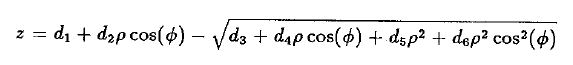where z is the sagitta coordinate of the surface that corresponds to a coordinate system. The origin of the coordinate system is observed at a point on the parent conic with coordinates (xo,0,zo). φ is the angular coordinate and ρ is the radial coordinate of the tilted cylindrical coordinate system. The tilt angle α, the parent conic and the point (xo,0,zo) are used to determine the constants from d1 to d6. Figure 1 shows the coordinate system.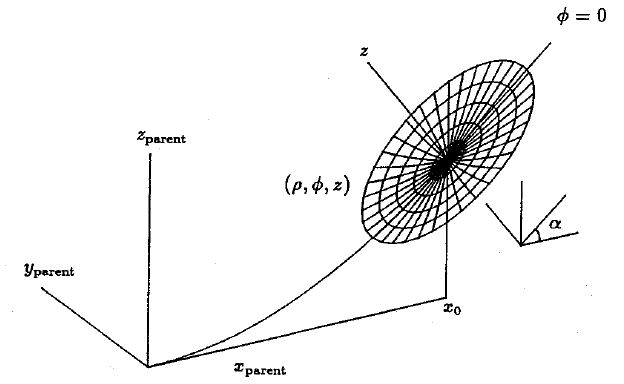Figure 1. Coordiante system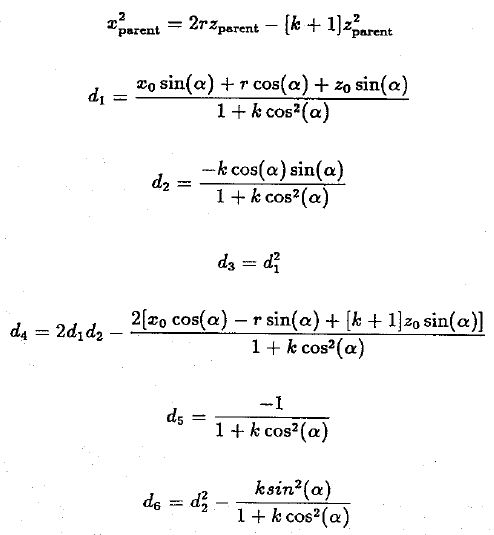The above equations define the exact shape of the off-axis conic surface. The surface geometry is separated into two coordinated machine motion sets, owing to the displacement limitations of the rapid tool servo motions. The two- axis orthogonal lathe motions are employed to produce the gross symmetric surface sagitta. By contrast, the tool servo motion is used to generate the non-rotationally symmetric geometry.

The applications of this technology to a large segmented astronomical mirror are shown in the below example illustrated in Figure 2. The parent surface is provided as a paraboloid of revolution. The optical speed of the parent mirror is f/2.5, with a 80 inch diameter and 200 inch focal length. The mirror includes 19 hexagonal segments along the optical axis. All the segments are divided into four parts: three expanding rows of six segments each and a center segment.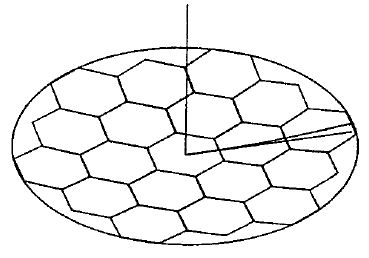Figure 2. Large segmented astronomical mirror

The non-rotationally symmetric concept can generate the total of 18 off-axis segments using a fast tool servo motion that is below 0.001 inches. However, there are two factors that reduce the required servo tool motions, one being the choice of α. Prior solutions for the parabolic case make use of the parent slope at (xo,0,zo). The fact that surface heights at φ = 0° and φ = 180° are found to be equal at maximum α accounts for the choice of α that minimizes servo motion.

The servo tool motion required for the circumscribed outermost segment upon subtracting the baseline motion from each point on a given ρ equal to the height at (z, ρ, φ = 0 ) is described in the wire grid illustration shown in Figure 3.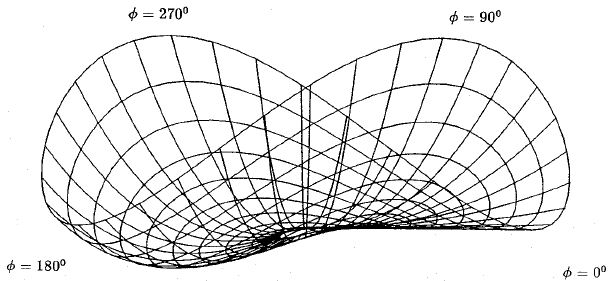Figure 3. Wire grid illustration of servo tool motion required for the circumscribed outermost segment

The subtraction of an optimum baseline is another factor that affects the total servo motion. The optimum baseline is chosen for each point on a given ρ, and its value represents the mean height extreme while φ moves forward from 0° to 360°. The two views of the servo tool motion subtracted with optimum baseline are shown in Figure 4.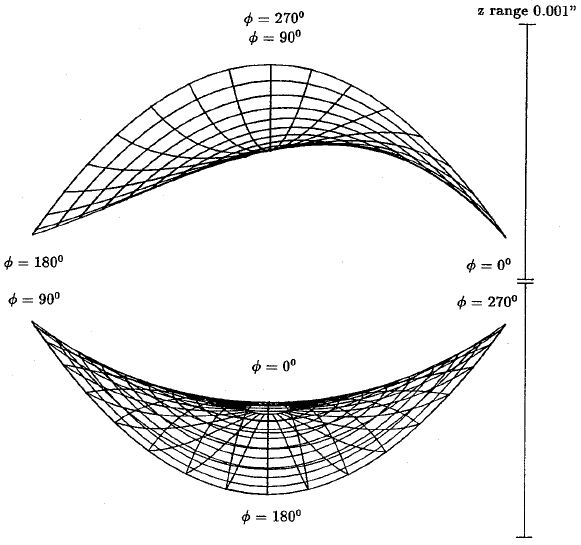Figure 4. Two views of the servo tool motion subtracted with optimum baseline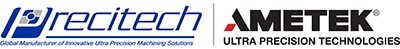This information has been sourced, reviewed and adapted from materials provided by Precitech.

## Citations

• APA

AMETEK Precitech. (2019, April 29). Far Off-Axis Conic Surfaces for Non-Rotationally Symmetric Surface Generation. AZoM. Retrieved on September 28, 2020 from https://www.azom.com/article.aspx?ArticleID=11921.

• MLA

AMETEK Precitech. "Far Off-Axis Conic Surfaces for Non-Rotationally Symmetric Surface Generation". AZoM. 28 September 2020. <https://www.azom.com/article.aspx?ArticleID=11921>.

• Chicago

AMETEK Precitech. "Far Off-Axis Conic Surfaces for Non-Rotationally Symmetric Surface Generation". AZoM. https://www.azom.com/article.aspx?ArticleID=11921. (accessed September 28, 2020).

• Harvard

AMETEK Precitech. 2019. Far Off-Axis Conic Surfaces for Non-Rotationally Symmetric Surface Generation. AZoM, viewed 28 September 2020, https://www.azom.com/article.aspx?ArticleID=11921.